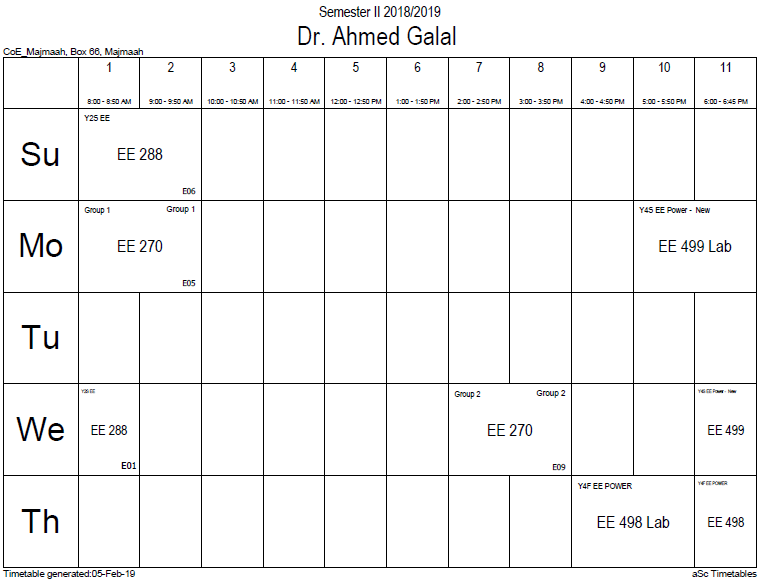## Euler's notation

Euler's notation uses a differential operator, denoted as D, which is prefixed to the function so that the derivatives of a function f are denoted by$Df ;$ for the first derivative,$D^2f ;$ for the second derivative, and$D^nf ;$ for the nth derivative, for any positive integer n.

When taking the derivative of a dependent variable y = f(x) it is common to add the independent variable x as a subscript to the D notation, leading to the alternative notation$D_x y ;$ for the first derivative,$D^2_x y;$ for the second derivative, and$D^n_x y ;$ for the nth derivative, for any positive integer n.

If there is only one independent variable present, the subscript to the operator is usually dropped, however.

Euler's notation is useful for stating and solving linear differential equations, as it simplifies presentation of the differential equation, which can make seeing the essential elements of the problem easier.

### Office HoursNo office hours### My Timetable### Contactsemail: [email protected]

Phone: 2570

### Welcome

Welcome To Faculty of Engineering### IEEEhttp://www.ieee.org/

/

### Bookmarkshttp://www.utk.edu/research/

http://science.doe.gov/grants/index.asp

http://www1.eere.energy.gov/vehiclesandfuels/

http://www.eere.energy.gov/

### Upcoming Conferences### Engineering quotes### Travel Web Siteshttp://www.hotels.com/

http://www.orbitz.com/

http://www.hotwire.com/us/index.jsp

http://www.kayak.com/

### Blackboardستقام اختبارات الميدتيرم يوم الثلاثاء 26-6-1440

حسب الجدول المعلن بلوحات الاعلان

### Summer trainingThe registration for summer training will start from 5th week of second semesterClass registration week 1

### برنامج التجسير### إحصائية الموقع

عدد الصفحات: 2879

البحوث والمحاضرات: 1280

الزيارات: 99470